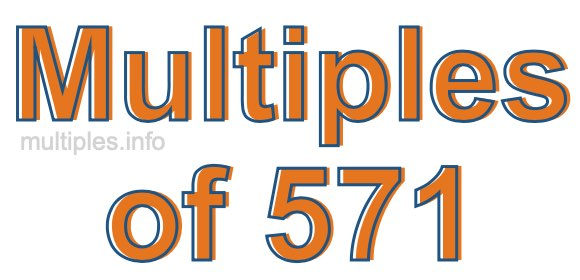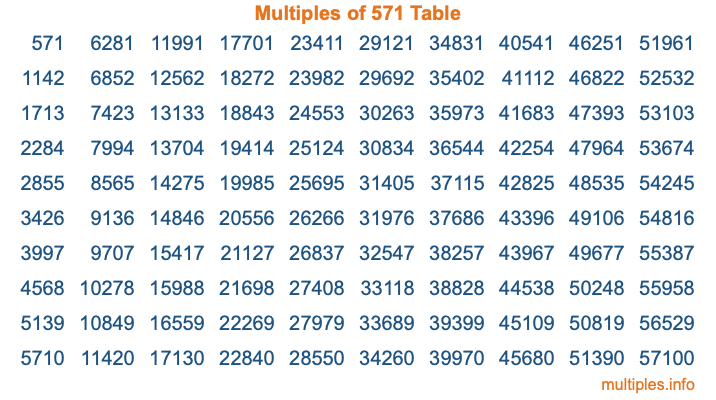Multiples of 571Welcome to the Multiples of 571 page. Here we will first teach you everything you will ever need to know about the multiples of 571, and then give you a study guide summary of everything we taught you to make sure you remember it all. Use this page to look up facts and learn information about the multiples of 571. This page will make you a multiples of five hundred seventy-one expert!

Definition of Multiples of 571
Multiples of 571 are all the numbers that when divided by 571 equal an integer. Each of the multiples of 571 are called a multiple. A multiple of 571 is created by multiplying 571 by an integer.

Therefore, to create a list of multiples of 571, you start with 1 multiplied by 571, then 2 multiplied by 571, then 3 multiplied by 571, and so on for as long as you want. Thus, the list of the first five multiples of 571 is 571, 1142, 1713, 2284, and 2855. To see a larger list of multiples of 571, see the printable image of Multiples of 571 further down on this page. We also have a category where you can choose any nth multiple of 571.

Multiples of 571 Checker
The Multiples of 571 Checker below checks to see if any number of your choice is a multiple of 571. In other words, it checks to see if there is any number (integer) that when multiplied by 571 will equal your number. To do that, we divide your number by 571. If the the quotient is an integer, then your number is a multiple of 571.

Is  a multiple of 571?

Least Common Multiple of 571 and ...
A Least Common Multiple (LCM) is the lowest multiple that two or more numbers have in common. This is also called the smallest common multiple or lowest common multiple and is useful to know when you are adding our subtracting fractions. Enter one or more numbers below (571 is already entered) to find the LCM.

Check out our LCM Calculator if you need more details about the Least Common Multiple or if you need the LCM for different numbers for adding and subtraction fractions.

nth Multiple of 571
As we stated above, 571 is the first multiple of 571, 1142 is the second multiple of 571, 1713 is the third multiple of 571, and so on. Enter a number below to find the nth multiple of 571.

th multiple of 571

Multiples of 571 vs Factors of 571
571 is a multiple of 571 and a factor of 571, but that is where the similarities end. All postive multiples of 571 are 571 or greater than 571. All positive factors of 571 are 571 or less than 571.

Below is the beginning list of multiples of 571 and the factors of 571 so you can compare:

Multiples of 571: 571, 1142, 1713, 2284, 2855, etc.

Factors of 571: 1, 571

As you can see, the multiples of 571 are all the numbers that you can divide by 571 to get a whole number. The factors of 571, on the other hand, are all the whole numbers that you can multiply by another whole number to get 571.

It's also interesting to note that if a number (x) is a factor of 571, then 571 will also be a multiple of that number (x).

Multiples of 571 vs Divisors of 571
The divisors of 571 are all the integers that 571 can be divided by evenly. Below is a list of the divisors of 571.

Divisors of 571: 1, 571

The interesting thing to note here is that if you take any multiple of 571 and divide it by a divisor of 571, you will see that the quotient is an integer.

Multiples of 571 Table
Below is an image of the first 100 multiples of 571 in a table. The table is in chronological order, column by column. The first column has the first ten multiples of 571, the second column has the next ten multiples of 571, and so on.The Multiples of 571 Table is also referred to as the 571 Times Table or Times Table of 571. You are welcome to print out our table for your studies.

Negative Multiples of 571
Although not often discussed or needed in math, it is worth mentioning that you can make a list of negative multiples of 571 by multiplying 571 by -1, then by -2, then by -3, and so on, to get the following list of negative multiples of 571:

-571, -1142, -1713, -2284, -2855, etc.

Multiples of 571 Summary
Below is a summary of important Multiples of 571 facts that we have discussed on this page. To retain the knowledge on this page, we recommend that you read through the summary and explain to yourself or a study partner why they hold true.

There are an infinite number of multiples of 571.

A multiple of 571 divided by 571 will equal a whole number.

571 divided by a factor of 571 equals a divisor of 571.

The nth multiple of 571 is n times 571.

The largest factor of 571 is equal to the first positive multiple of 571.

571 is a multiple of every factor of 571.

571 is a multiple of 571.

A multiple of 571 divided by a divisor of 571 equals an integer.

571 divided by a divisor of 571 equals a factor of 571.

Any integer times 571 will equal a multiple of 571.

Multiples of a Number
Here you can get the multiples of another number, all with the same attention to detail as we did for multiples of 571 on this page.

Multiples of
Multiples of 572
Did you find our page about multiples of five hundred seventy-one educational? Do you want more knowledge? Check out the multiples of the next number on our list!